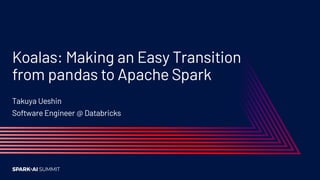Se está descargando tu SlideShare. ×

# Koalas: Making an Easy Transition from Pandas to Apache Spark

Anuncio
Anuncio
Anuncio
Anuncio
Anuncio
Anuncio
Anuncio
Anuncio
Anuncio
Anuncio
AnuncioCargando en…3
×

1 de 26 Anuncio

# Koalas: Making an Easy Transition from Pandas to Apache Spark

Koalas is an open-source project that aims at bridging the gap between big data and small data for data scientists and at simplifying Apache Spark for people who are already familiar with pandas library in Python. Pandas is the standard tool for data science and it is typically the first step to explore and manipulate a data set, but pandas does not scale well to big data.

Koalas is an open-source project that aims at bridging the gap between big data and small data for data scientists and at simplifying Apache Spark for people who are already familiar with pandas library in Python. Pandas is the standard tool for data science and it is typically the first step to explore and manipulate a data set, but pandas does not scale well to big data.

Anuncio
Anuncio

### Koalas: Making an Easy Transition from Pandas to Apache Spark

1. 1. Koalas: Making an Easy Transition from pandas to Apache Spark Takuya Ueshin Software Engineer @ Databricks
2. 2. About Takuya Ueshin Software Engineer at Databricks ▪ Apache Spark committer and PMC member ▪ Focusing on Spark SQL and PySpark ▪ Koalas maintainer
3. 3. Outline ▪ What’s Koalas? ▪ pandas vs Apache Spark at a high level ▪ Koalas 1.0 ▪ Demo ▪ InternalFrame ▪ Index and Default Index ▪ Roadmap
4. 4. What’s Koalas? ▪ Announced April 24, 2019 ▪ Aims at providing the pandas API on top of Apache Spark ▪ Unifies the two ecosystems with a familiar API ▪ Seamless transition between small and large data ▪ For pandas users ▪ Scale out the pandas code using Koalas ▪ Make learning PySpark much easier ▪ For PySpark users ▪ More productive by pandas-like functions
5. 5. pandas ▪ Authored by Wes McKinney in 2008 ▪ The standard tool for data manipulation and analysis in Python ▪ The current version: 1.0.4 Stack Overflow Trends
6. 6. pandas ▪ Deeply integrated into Python data science ecosystem ▪ numpy ▪ matplotlib ▪ scikit-learn ▪ Can deal with a lot of different situations, including: ▪ Basic statistical analysis ▪ Handling missing data ▪ Time series, categorical variables, strings
7. 7. Apache Spark ▪ De facto unified analytics engine for large-scale data processing ▪ Streaming ▪ ETL ▪ ML ▪ Originally created at UC Berkeley by Databricks’ founders ▪ PySpark API for Python; also API support for Scala, R and SQL ▪ The latest version: 3.0.0
8. 8. pandas DataFrame PySpark DataFrame Column df['col'] df['col'] Mutability Mutable Immutable Execution Eagerly Lazily Add a column df['c'] = df['a'] + df['b'] df = df.withColumn('c', df['a'] + df['b']) Rename columns df.columns = ['a','b'] df = df.select(df['c1'].alias('a'), df['c2'].alias('b')) df = df.toDF('a', 'b') Value count df['col'].value_counts() df.groupBy(df['col']).count() .orderBy('count', ascending=False) pandas DataFrame vs. PySpark DataFrame
9. 9. A short example import pandas as pd df = pd.read_csv("/path/to/my_data.csv") df.columns = ['x', 'y', 'z1'] df['x2'] = df.x * df.x df = (spark.read .option("inferSchema", "true") .csv("/path/to/my_data.csv")) df = df.toDF('x', 'y', 'z1') df = df.withColumn('x2', df.x * df.x) PySparkpandas
10. 10. A short example import pandas as pd df = pd.read_csv("/path/to/my_data.csv") df.columns = ['x', 'y', 'z1'] df['x2'] = df.x * df.x import databricks.koalas as ks df = ks.read_csv("/path/to/my_data.csv") df.columns = ['x', 'y', 'z1'] df['x2'] = df.x * df.x Koalaspandas